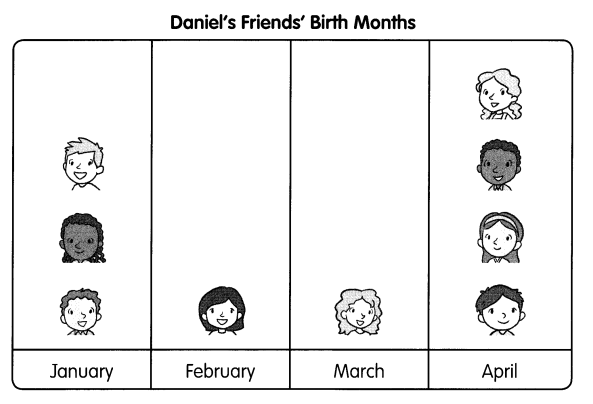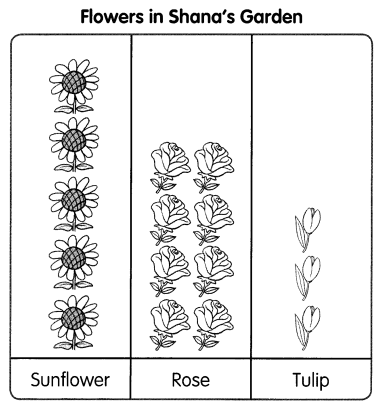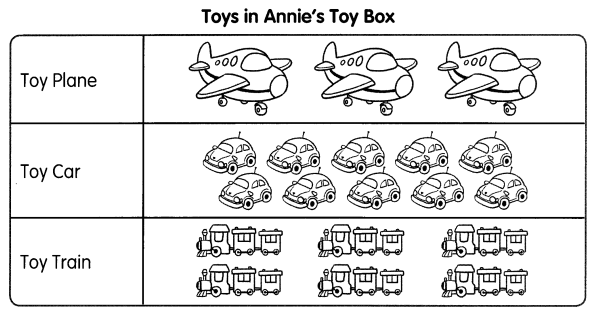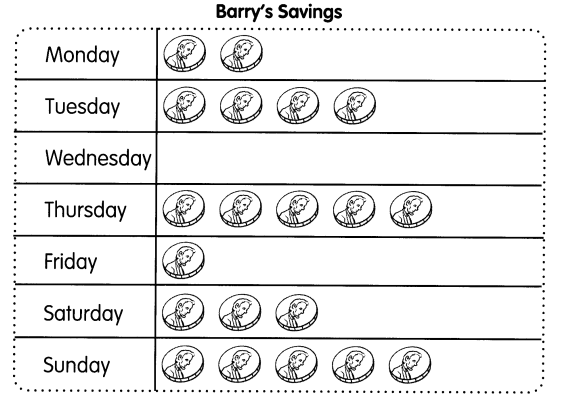# Math in Focus Grade 1 Chapter 11 Practice 1 Answer Key Simple Picture Graphs

Practice the problems of Math in Focus Grade 1 Workbook Answer Key Chapter 11 Practice 1 Simple Picture Graphs to score better marks in the exam.

## Math in Focus Grade 1 Chapter 11 Practice 1 Answer Key Simple Picture Graphs

Daniel draws a picture graph of his friends’ birth months.Use the graph to answer the questions.

Question 1.
How many friends are born in January? ____
Answer: 3 friends are born in January

Question 2.
In which month are most of his friends born? ____
Answer: Most of the friends are born on the month of April

Look at the picture graph. Then fill in the blanks.

This picture graph shows the flowers in Shana’s garden.Question 3.
There are ___ sunflowers.

Question 4.
There are ___ roses.

Question 5.
There are ____ tulips.

Question 6.
There are ___ more roses than tulips.
Answer: There are 5 more roses than tulips.

Question 7.
There are ____ fewer tulips than sunflowers.
Answer: There are 3 fewer tulips than sunflowers.

Question 8.
There are ___ flowers in all.
Answer: There are 17 flowers in all.

Look at the picture graph. Then fill in the blanks.

This picture graph shows all the toys in Annie’s toy box.Question 9.
There are ___ toy planes ¡n Annie’s box.
Answer: There are 3 toy planes ¡n Annie’s box.

Question 10.
There are ___ toy cars.
Answer: There are 10 toy cars.

Question 11.
There are ___ toy trains.
Answer: There are 6 toy trains.

Question 12.
The number of ___________ is the greatest.
Answer: The number of toy car is the greatest.

Question 13.
The number of ___________ is the least.
Answer: The number of toy planes is the least.

Question 14.
Annie has ___ toys in all.
Answer: Annie has 19 toys in all.

Question 15.
There are ________ more toy cars than toy trains.
Answer: There are 4 more toy cars than toy trains.

Question 16.
There are 7 fewer ____________ than ________
Answer: There are 7 fewer toy planes than toy cars

Look at the picture graph. Then fill in the blanks.

This picture graph shows the coins Barry saves in a week.Question 17.
How many coins does Barry save on Monday? ___ coins
Answer: Barry save 2 coins on Monday

Question 18.
How many coins does he save on Tuesday? ___ coins
Answer: Barry save 4 coins on Tuesday

Question 19.
Barry saves 1 coin on Friday.
He saves ___ more coins on Saturday.
Answer: He saves 2 more coins on Saturday.

Question 20.
How many coins in all does he save from Thursday to Saturday? ___ coins
Answer: From Thursday to Saturday Barry has 17 coins in all

Question 21.
He does not save on ____
Answer: He does not save on Wednesday